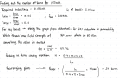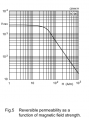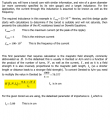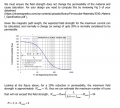# finding the number of turns for my inductance.

#### HappyC4mper

Joined Oct 13, 2017
71
Hey guys, so i've calculated that I need an inductance of 0.150mH for my inductor. However, I'm trying to figure out the number of turns I need for it. #
I've got an answer of 24 turns but this doesn't feel right to me. Could someone double check my calculations?below is the datasheet for the toroid I used as well as the graph(from the datasheet) I used.
Datasheet for toroidIf anyone is interested in the example I was using to calculate thisMany thanks!

Last edited:

#### BobTPH

Joined Jun 5, 2013
3,475
If I am following your post correctly, the calculation you are showing is for the max number of turns you can have without saturating the core. But your question was about how many turns are required to get 150uH of inductance. This is an entirely different calculation, no?

Of course, you need to do both, and if the second calculation exceeds the first, then the core is not appropriate for the specs you want. The equation for the inductance should have N^2 it in.

Bob

#### ci139

Joined Jul 11, 2016
1,696